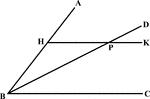### Angle Bisector Drawn Parallel to A Side

Illustration to show that if through any point in the bisector of an angle a line is drawn parallel…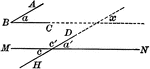### Angles With Parallel Sides

Illustration of two angles whose sides are parallel, each to each, to show they are either equal or…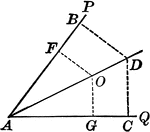### Angle Bisector

Illustration showing that the bisector of a given angle is the locus of points equidistant from the…### Perpendicular Bisector of a Line

Illustration showing that the perpendicular bisector of a given line is the locus of points equidistant…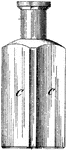### Four Sided Bottle

This four sided bottle is a rigid container. A glass bottle is a bottle created from glass. Glass bottles…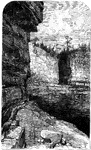### Cliff

An illustration of a cliff located in the Adirondacks.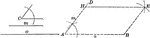### Construction of a Parallelogram When Given Two Sides and Included Angle

Illustration of the construction used to make a parallelogram when given two sides and the included…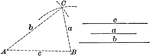### Construction of a Triangle When Given Three Sides

Illustration of the construction used to make a triangle when given three sides.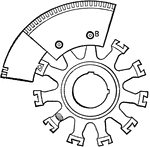### Armature core

"Side view of built up armature core. The sheet metal ring sections containing the teeth are fastened…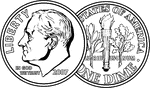### Both sides of a Dime

An illustration of both sides of a U.S. Dime.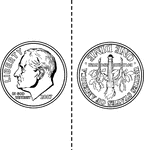### Two Sided Dime

An illustration of both sides of a U.S. Dime that can be cutout and folded.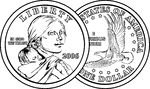### Both sides of a Dollar

An illustration of both sides of a U.S. Dollar Coin.### Two Sided Dollar

An illustration of both sides of a U.S. Dollar Coin that can be cutout and folded.### Four Pole Machine

"Partial sketch of a four pole machine laid on its side." -Hawkins, 1917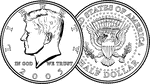### Both sides of a Half Dollar

An illustration of both sides of a U.S. Half Dollar.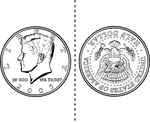### Two Sided Half Dollar

An illustration of both sides of a U.S. Half Dollar that can be cutout and folded.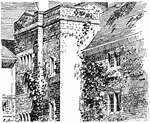### House (Side)

An illustration of the side of a large house.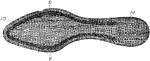### Shoe Insole

An extra strip of material put inside a shoe for comfort or protection.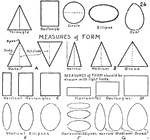### Measures of Form

Labeled shapes showing various measures of form.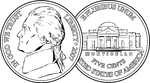### Both sides of a Nickel

An illustration of both sides of a U.S. nickel.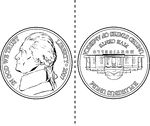### Two Sided Nickel

An illustration of both sides of a U.S. nickel that can be cutout and folded.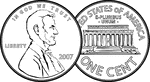### Both sides of a Penny

An illustration of both sides of a U.S. Penny.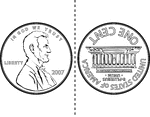### Two Sided Penny

An illustration of both sides of a U.S. Penny that can be cutout and folded.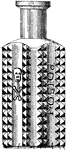### Four Sided Poison Bottle

This bottle was intended to hold poison. Poisons are substances that can cause disturbances to organisms,…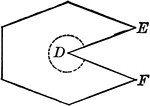### Concave Polygon

Illustration of a concave polygon. A concave polygon is a polygon of which tow or more sides, if produced,…### Convex Polygon

Illustration of a convex polygon. A polygon is convex when no side when produced will enter the polygon.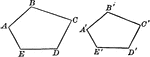### Similar Polygons (Pentagons) That Can Be Used To Show Proportionality

Illustration that shows similar polygons (pentagons) that can be used to show proportionality.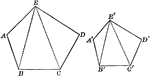### Similar Polygons (Pentagons) That Can Be Used To Show Proportionality

Illustration that shows similar polygons (pentagons) that can be used to show proportionality.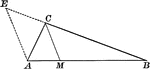### Angle Bisector of Triangle Dividing Opposite Side Into Proportionate Segments

Illustration to show that an angle bisector of a triangle divides the opposite side into segments which…### Exterior Angle Bisector of Triangle Dividing Opposite Side Into Proportionate Segments

Illustration to show that a bisector of a an exterior angle of a triangle divides the opposite side…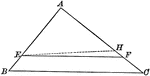### Triangle Divided by Parallel Lines to Form Proportionate Triangles

Illustration to show that if a line divides two sides of a triangle proportionally, it is parallel to…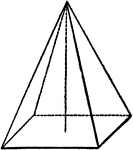### Pryamid

"A pyramid is a solid whose base is a polygon, and whose sides are triangles uniting at a common point,…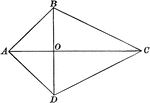Illustration of a quadrilateral which has two adjacent sides equal and the other two sides equal. It…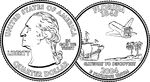### Both sides of a Quarter

An illustration of both sides of a U.S. Quarter.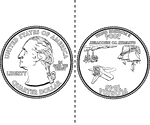### Two Sided Quarter

An illustration of both sides of a U.S. Quarter that can be cutout and folded.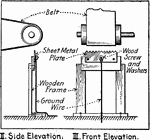### Using a Grounded Metallic Comb to Reduce Static Charge, Front and Side View

"Method of using a grounded metallic comb near the driving belt to relieve the belt of static charges."…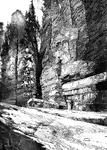### Table Rock

Historically, the name referred to a large shelf of rock which jutted out from the Canadian shore, located…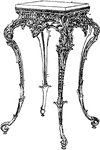### Intrigue Design Table

A table is an item of furniture comprising an open, flat surface supported by a base or legs. It may…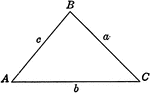### Triangle

Triangle with sides a, b, c and angles A, B, C labeled.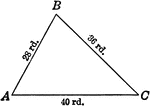### Triangle 28, 36, 40

Triangle with sides labeled with numerals 28, 36, 40 and angles A, B, C labeled.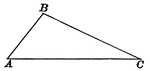### Triangle ABC

Illustration showing a triangle with angles A, B, and C labeled.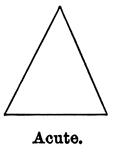### Acute Triangle

Illustration showing an acute triangle (one that has all three angles that are acute).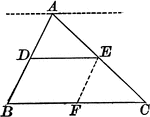### Triangle With Bisector Parallel to the Base

Illustration of a triangle showing that when a line parallel to the base of a triangle bisects one side,…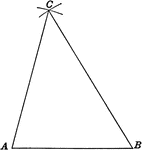### Triangle Construction When Given 2 Angles and Included Side

Triangle construction when given two angles and the included side.### Equiangular Triangle

Illustration showing an equiangular triangle (one that has all three angles that are equal).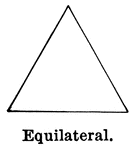### Equilateral Triangle

Illustration showing an equilateral triangle (one that has three sides equal).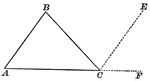### Triangle with Exterior Angles Drawn

Illustration showing a triangle with exterior angles. This can be used to show the sum of the three…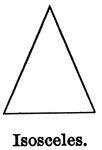### Isosceles Triangle

Illustration showing an isosceles triangle (one that has two sides equal).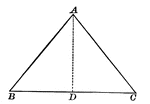### Isosceles Triangle With Interior Segment Drawn

Illustration showing an isosceles triangle with a segment inside. This can be used to show that in an…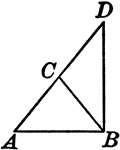### Leg Produced through the Vertex of an Isosceles Triangle

Illustration to show that if one of the legs of an isosceles triangle is produced through the vertex…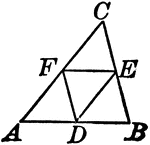### Midpoints of Triangle Divide Triangle into Four Equal Triangles

Illustration to show that if the lines joining the middle points of the sides of a triangle divide the…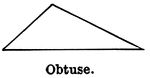### Obtuse Triangle

Illustration showing an obtuse triangle (one that has one obtuse angle).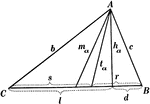### Parts Of A Triangle

Illustration used to show the various parts of a triangle: sides, angles, medians, altitudes, bisectors,…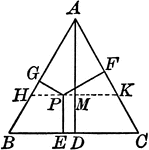### Perpendiculars Dropped Any Point in the Equilateral Triangle

Illustration to show the perpendiculars dropped from any point in the equilateral triangle to the three…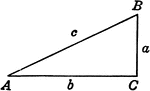### Right Triangle ABC

Right triangle with sides a, b, c and angles A, B, C labeled.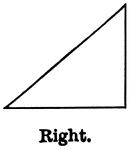### Right Triangle

Illustration showing a right triangle (one that has one right angle).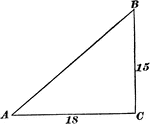### Right Triangle With Sides 15 and 18

Right triangle with sides 15 and 18.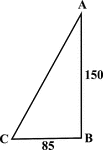### Right Triangle With Sides 85 and 150

Illustration of a right triangle with legs labeled 150 and 85. This could be used to find the hypotenuse…